# Capitalize First Letter – Excel & Google Sheets

This tutorial will teach you how to capitalize first letter(s) in Excel and Google Sheets.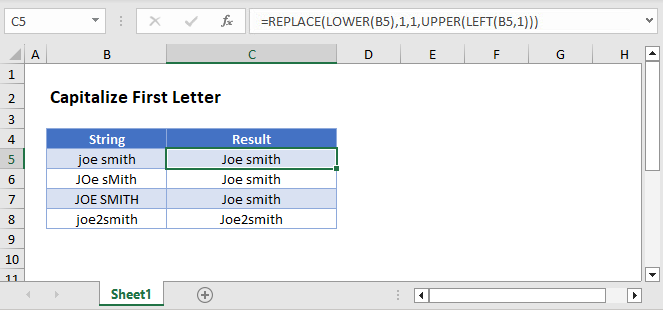## Capitalize First Letter of All Words

The simplest way to capitalize first letters in a text string is to use the PROPER Function.

``=PROPER(B3)``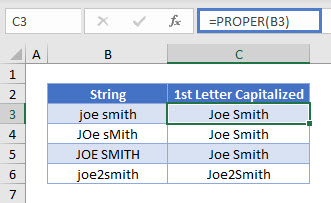The PROPER Function capitalizes the first letter in every word in the text string and convers all other letters to lower case.

Note: The PROPER Function treats any numeric character like a space. So “joe2smith” was changed to “Joe2Smith”.

## Capitalize First Letter Only

Instead, to capitalize only the first letter of your text string, use the REPLACE function along with the UPPER and LEFT functions.

``=REPLACE(B3,1,1,UPPER(LEFT(B3,1)))``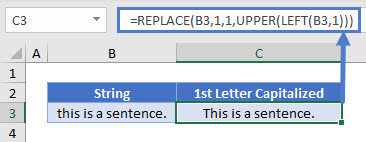The REPLACE Function simply replaces the first character with a capitalized version of the first character.

You can add in the LOWER Function to set all other characters to lower case:

``=REPLACE(LOWER(B3),1,1,UPPER(LEFT(B3,1)))``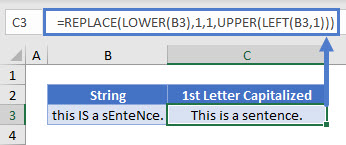## Capitalize First Letter in Google Sheets

These formulas work exactly the same in Google Sheets as in Excel.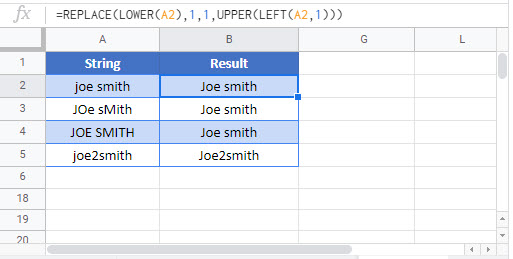### Excel Practice Worksheet

Practice Excel functions and formulas with our 100% free practice worksheets!